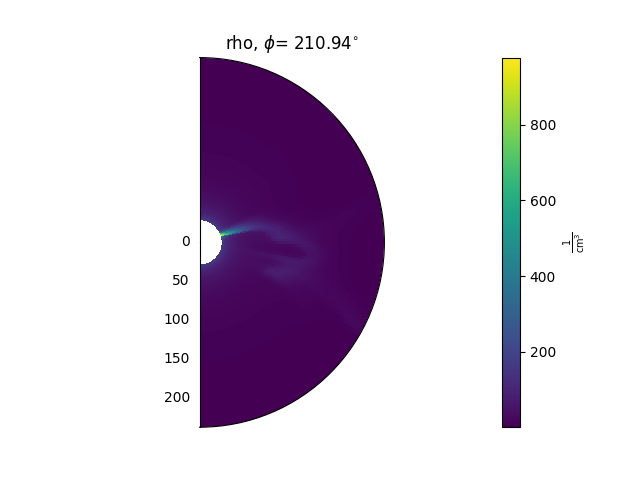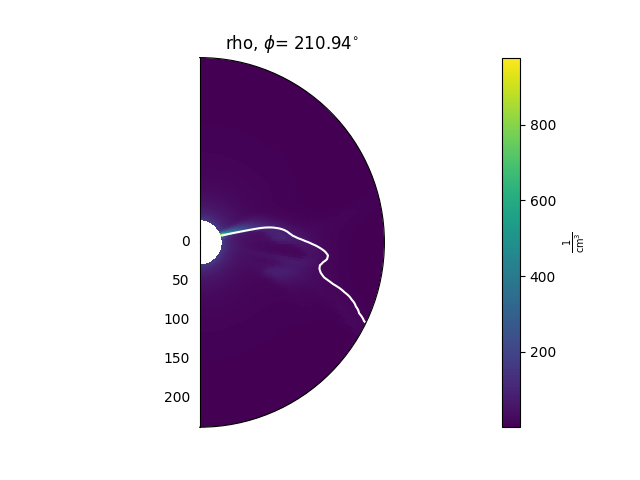Getting started

psipy is a package for loading and visualising the output of PSI’s MAS model runs. This page provides some narrative documentation that should get you up and running with obtaining, loading, and visualising some model results.

Getting data

The PSI MHDWeb pages give access to MAS model runs. The runs are indexed by Carrington rotation, and for each Carrington rotation there are generally a number of different runs, varying in the type model run and/or the boundary conditions.

psipy stores the output variables from a single MAS run in the MASOutput object. To create one of these, specify the directory which has all of the output .hdf files you want to load:

from psipy.model import MASOutput

directory = '/path/to/files'
mas_output = MASOutput(directory)

It is assmumed that the files have the filename structure '{var}{timestep}.hdf', where var is a variable name, and timestep is a three-digit zero-padded integer timestep.

To see which variables have been loaded, we can look at the .variables attribute:

print(mas_output.variables)

This will print a list of the variables that have been loaded. Each individual variable can then be accessed with square brackets, for example to get the radial magnetic field component:

br = mas_output['br']

This will return a Variable object, which stores the underlying data as a xarray.DataArray under the Variable.data property.

Data coordinates

The data stored in Variable.data contains the values of the data as a normal array, and in addition stores the coordinates of each data point.

MAS model outputs are defined on a 3D grid of points on a spherical grid. The coordinate names are 'r', 'theta', 'phi'. The coordinate values along each dimension can be accessed using the r_coords, theta_coords, phi_coords properties, e.g.:

rvals = br.r_coords

Plotting data

Variable objects have methods to plot 2D slices of the data. These methods are:

A typical use looks like this:

ax = plt.subplot(1, 1, 1, projection='polar')
model['rho'].plot_phi_cut(index, ax=ax, ...)

and produces an output like this:For more examples of how to use these methods, see the Examples gallery.

Animating timesteps

If multiple timesteps have been loaded, each of the above methods can either be used to plot animations over time, or single time slices. By default they will produce animations which then need to be manually saved. As an example, to create and save an animated cut in the phi direction:

animation = model['rho'].plot_phi_cut(phi_index, ...)
animation.save('my_animation.mp4')

Contouring data

There are also methods that can be used to plot contours of the data on top of 2D slices. As an example, this can be helpful for plotting the heliospheric current sheet, by contouring $$B_{r} = 0$$. These methods are

A typical use looks like this:

ax = plt.subplot(1, 1, 1, projection='polar')
model['rho'].plot_phi_cut(index, ax=ax, ...)
model['br'].contour_phi_cut(index, levels=, ax=ax, ...)

and produces outputs like this:For more examples of how to use these methods, see the Examples gallery.

Normalising data before plotting

Sometimes it is helpful to multiply data by an expected radial falloff, e.g. multiplying the density by $$r^{2}$$. This can be done using the Variable.radial_normalized method, e.g.:

rho = mas_output['rho']